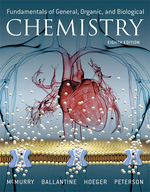×
Get Full Access to Fundamentals Of General, Organic, And Biological Chemistry (Mastering Chemistry) - 8 Edition - Chapter 7 - Problem 7.56
Get Full Access to Fundamentals Of General, Organic, And Biological Chemistry (Mastering Chemistry) - 8 Edition - Chapter 7 - Problem 7.56

×ISBN: 9780134015187 2044

## Solution for problem 7.56 Chapter 7

Fundamentals of General, Organic, and Biological Chemistry (Mastering Chemistry) | 8th Edition

• Textbook Solutions
• 2901 Step-by-step solutions solved by professors and subject experts
• Get 24/7 help from StudySoup virtual teaching assistantsFundamentals of General, Organic, and Biological Chemistry (Mastering Chemistry) | 8th Edition

4 5 1 349 Reviews
28
0
Problem 7.56

(a) $$\left[\mathrm{O}_{2}\right]$$ at equilibrium when $$\left[\mathrm{CO}_{2}\right]=0.18 \mathrm{~mol} / \mathrm{L}$$ and [CO]=0.0200 mol/L

(b) $$\left[\mathrm{CO}_{2}\right]$$ at equilibrium when [CO]=0.080 mol/L and $$\left[\mathrm{O}_{2}\right]=0.520 \mathrm{~mol} / \mathrm{L}$$

Text Transcription:

[O_2]

[CO_2]=0.18 mol/L

[CO_2]

[O_2]=0.520 mol/ L

Step-by-Step Solution:
Step 1 of 3

Chapter 5: Chemical bonding I 1. Bond characteristics - Pure (nonpolar bond) covalent bond: electrons shared equally - Polar covalent bond: electrons shared unequally - Ionic Bond: electrons transferred 2. VSEPR - Basis: pairs of valence electrons in bonded atoms repel one another 3. States of matter - Gas molecules move with great energy >>> overcome intermolecular force >> intermolecular force of little importance 1. Intermolecular forces - Determine melting – freezing points and other physical properties - Types of intermolecular forces: + Dispersion/London Force + Dipole – Dipole + H-Bonding + Ion – Dipole - More electrons >> higher molecular weight >>> Stronger interactions >>>> Higher boiling points. a. Dispersion - Attraction between positive + and negative – ends of nonpermanent dipoles - All molecules have Dispersion forces - For same number of e/molecular weight >>>polar molecules have higher boiling point than nonpolar ones <<<< stronger intermolecular forces in Polar molecules b. Dipole – Dipole - Dipole – Dipole >> Dispersion Because Dipole – Dipole has permanent dipoles - Polar molecules have permanent dipoles - Dipole – Dipole only exists in Polar molecules, not in Nonpolar. c. Hydrogen bond - Hydrogen bond is a type of Dipole – Dipole bond - Hydrogen bonds need: + Partially positive H atom + lone e pair on small electronegative ato

Step 2 of 3

Step 3 of 3

##### ISBN: 9780134015187

Fundamentals of General, Organic, and Biological Chemistry (Mastering Chemistry) was written by Aimee Notetaker and is associated to the ISBN: 9780134015187. The full step-by-step solution to problem: 7.56 from chapter: 7 was answered by Aimee Notetaker, our top Chemistry solution expert on 04/25/22, 03:57PM. This full solution covers the following key subjects: . This expansive textbook survival guide covers 281 chapters, and 240 solutions. Since the solution to 7.56 from 7 chapter was answered, more than 204 students have viewed the full step-by-step answer. The answer to “?Use your answer from 7.54 to calculate the following:(a) $$\left[\mathrm{O}_{2}\right]$$ at equilibrium when $$\left[\mathrm{CO}_{2}\right]=0.18 \mathrm{~mol} / \mathrm{L}$$ and [CO]=0.0200 mol/L(b) $$\left[\mathrm{CO}_{2}\right]$$ at equilibrium when [CO]=0.080 mol/L and $$\left[\mathrm{O}_{2}\right]=0.520 \mathrm{~mol} / \mathrm{L}$$Text Transcription:[O_2] [CO_2]=0.18 mol/L[CO_2] [O_2]=0.520 mol/ L” is broken down into a number of easy to follow steps, and 37 words. This textbook survival guide was created for the textbook: Fundamentals of General, Organic, and Biological Chemistry (Mastering Chemistry), edition: 8.

Unlock Textbook Solution